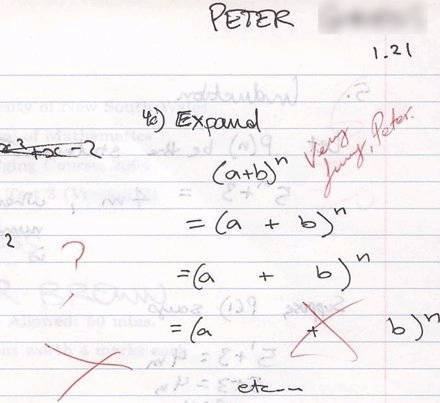SEARCH HOMEMath Central Quandaries & QueriesQuestion from Saif, a student: how would you expand (a^4 - b^4) ???Hi Saif,

You can check by multiplication that (x+y)(x-y) = x2 - y2. This means that whenever you see a difference of two squares, as on the right hand side, you can replace it by what's on the left hand side.

From the rules of exponents, any base to an even power is the square of the base to half that power. For example, c6 = (c3)2.

You equation is (a2)2 - (b2)2, a difference of two squares. Now use the results from the first paragraph, and then use them a second time on part of what you get.

Victoria

Saif,

Victoria's answer is a good one. Don't make the mistake that Peter made in a similar question (see below).:)
Stephen.Math Central is supported by the University of Regina and The Pacific Institute for the Mathematical Sciences.